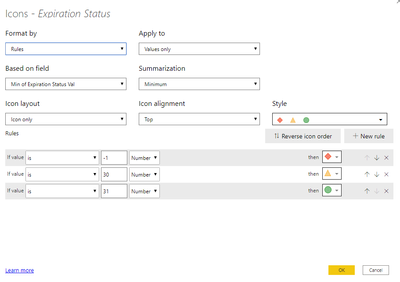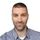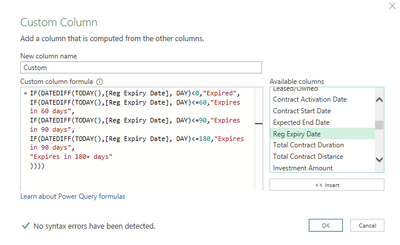cancel
Showing results for
Did you mean:

Fabric is Generally Available. Browse Fabric Presentations. Work towards your Fabric certification with the Cloud Skills Challenge.Helper IV

## Measure expire date

I have table with expite date for each products.

I wanted to make a conditional column with icon that shows those product under 30 days that expire it gonna have yellow icon and the less than 0 gonna be red icon and those products that have over 30 days expieration gonna have green icon with this measure

Expiration Status Val =
IF([ExpiryDate] < TODAY(), -1
, IF([ExpiryDate] > TODAY(), 31
, 30
)
)

But it dosen't seems work for the yellow icon, yellow appear only if it will be expire today. How can I fix it?2 ACCEPTED SOLUTIONSMemorable Member

@selected_ ,

Conditional formatting is correct.
In my opinion, you need to create different logic for calculated field.

Try this:

Expiration Status Val final =
IF(DATEDIFF(TODAY(),'Table'[Date], DAY)<0,1,  -- if expiration date is in the past return 1
IF(DATEDIFF(TODAY(),'Table'[Date], DAY)<30,30, -- if expiration date is in next 30 days return 30
31) -- else return 31
)Memorable Member

@selected_ ,

Here are some examples:

Expiration Category v2 =
IF(DATEDIFF(TODAY(),'Table'[Date], DAY)<0,"Expired",
IF(DATEDIFF(TODAY(),'Table'[Date], DAY)<=30,"Expires in 30 days",
IF(DATEDIFF(TODAY(),'Table'[Date], DAY)<=60,"Expires in 60 days",
IF(DATEDIFF(TODAY(),'Table'[Date], DAY)<=180,"Expires in 180 days",
"Expires in 180+ days"
))))

----

Expiration category =
IF(
AND(DATEDIFF(TODAY(),'Table'[Date], DAY)>0,DATEDIFF(TODAY(),'Table'[Date], DAY)<=30),"30 days",
IF(AND(DATEDIFF(TODAY(),'Table'[Date], DAY)>30,DATEDIFF(TODAY(),'Table'[Date], DAY)<=60),"60 days",
IF(AND(DATEDIFF(TODAY(),'Table'[Date], DAY)>60,DATEDIFF(TODAY(),'Table'[Date], DAY)<=180),"180 days"
)
)
)

---

Add a measure which will count number of products:
Countrows = COUNTROWS('Table')

Use one of first 2 calculations as calculated columns and use last one as measure.
6 REPLIES 6Helper I

@nandic This is great example for my case too.  However, I also have the blank value in date field.  How is the formula should I add to check blank value.  I added one line below (in red) but doesn't work.

Expiration Category v2 =
IF(DATEDIFF(TODAY(),'Table'[Date], DAY)<0,"Expired",
IF(DATEDIFF(TODAY(),'Table'[Date], DAY)<=30,"Expires in 30 days",
IF(DATEDIFF(TODAY(),'Table'[Date], DAY)<=60,"Expires in 60 days",
IF(DATEDIFF(TODAY(),'Table'[Date], DAY)<=180,"Expires in 180 days",
IF('Table'[Date] = BLANK (), "N/A",
"Expires in 180+ days"
)))))Anonymous
Not applicable

i'm getting the same error.Memorable Member

@selected_ ,

Conditional formatting is correct.
In my opinion, you need to create different logic for calculated field.

Try this:

Expiration Status Val final =
IF(DATEDIFF(TODAY(),'Table'[Date], DAY)<0,1,  -- if expiration date is in the past return 1
IF(DATEDIFF(TODAY(),'Table'[Date], DAY)<30,30, -- if expiration date is in next 30 days return 30
31) -- else return 31
)Helper IV

Thanks it worked. Is that possible to make another measure on amount product will expire within 30 days and 60 days and 180 days?Memorable Member

@selected_ ,

Here are some examples:

Expiration Category v2 =
IF(DATEDIFF(TODAY(),'Table'[Date], DAY)<0,"Expired",
IF(DATEDIFF(TODAY(),'Table'[Date], DAY)<=30,"Expires in 30 days",
IF(DATEDIFF(TODAY(),'Table'[Date], DAY)<=60,"Expires in 60 days",
IF(DATEDIFF(TODAY(),'Table'[Date], DAY)<=180,"Expires in 180 days",
"Expires in 180+ days"
))))

----

Expiration category =
IF(
AND(DATEDIFF(TODAY(),'Table'[Date], DAY)>0,DATEDIFF(TODAY(),'Table'[Date], DAY)<=30),"30 days",
IF(AND(DATEDIFF(TODAY(),'Table'[Date], DAY)>30,DATEDIFF(TODAY(),'Table'[Date], DAY)<=60),"60 days",
IF(AND(DATEDIFF(TODAY(),'Table'[Date], DAY)>60,DATEDIFF(TODAY(),'Table'[Date], DAY)<=180),"180 days"
)
)
)

---

Add a measure which will count number of products:
Countrows = COUNTROWS('Table')

Use one of first 2 calculations as calculated columns and use last one as measure.Helper I

Hi Nandic

I tried using your solution and Power Query is throwing me an error. I created a new custom column and entered this FxOn executing it, it throws an error "Expression.Error: The name 'IF' wasn't recognized. Make sure it's spelled correctly."Announcements#### Power BI Monthly Update - November 2023

Check out the November 2023 Power BI update to learn about new features.#### Fabric Community News unified experience

Read the latest Fabric Community announcements, including updates on Power BI, Synapse, Data Factory and Data Activator.#### The largest Power BI and Fabric virtual conference

130+ sessions, 130+ speakers, Product managers, MVPs, and experts. All about Power BI and Fabric. Attend online or watch the recordings.Top Solution Authors
Top Kudoed Authors
Users online (4,286)